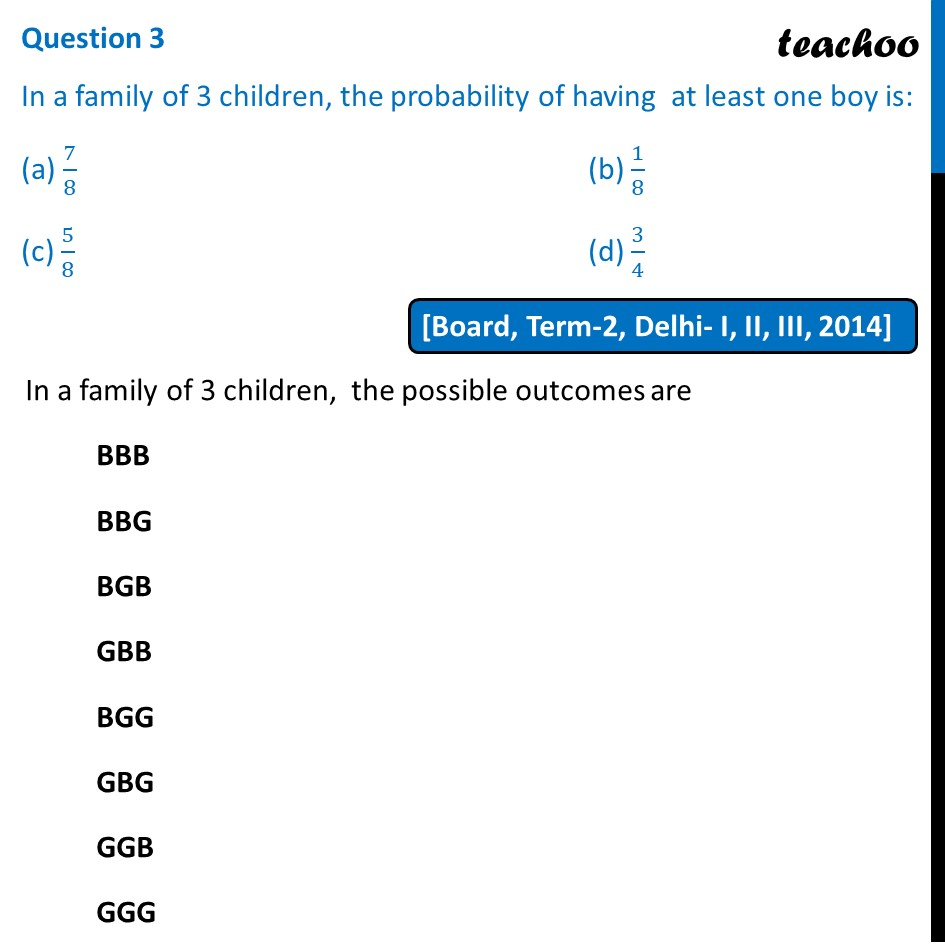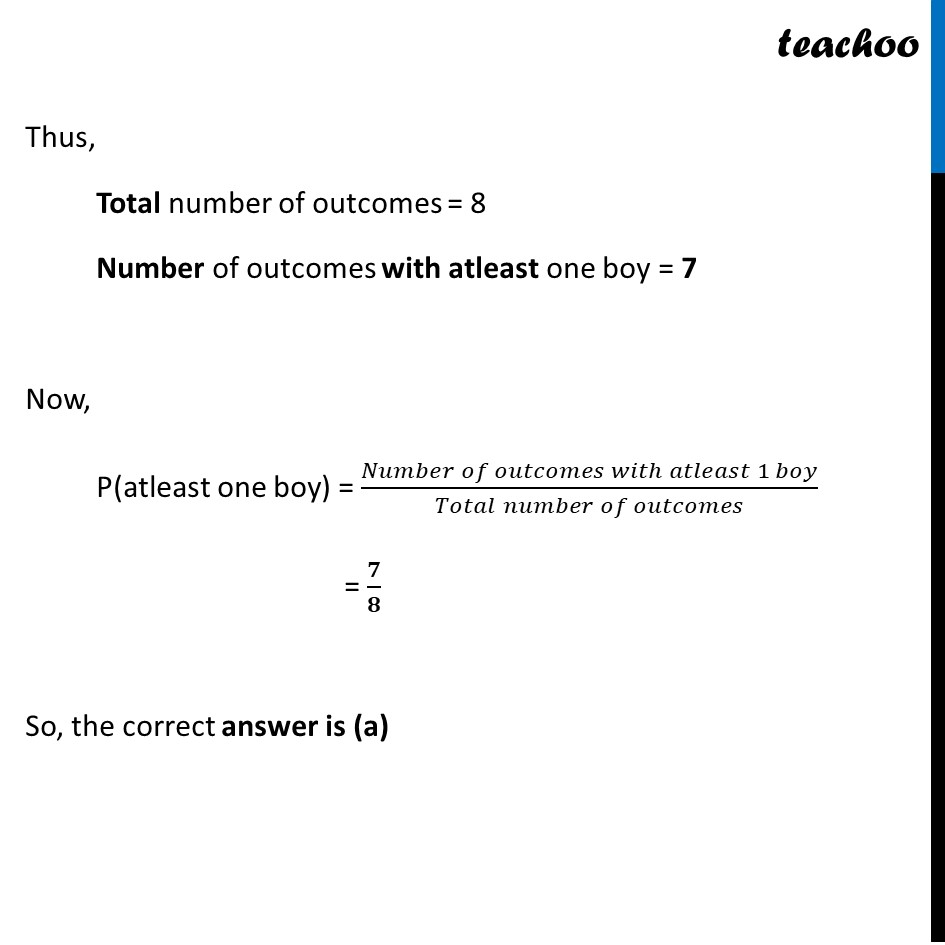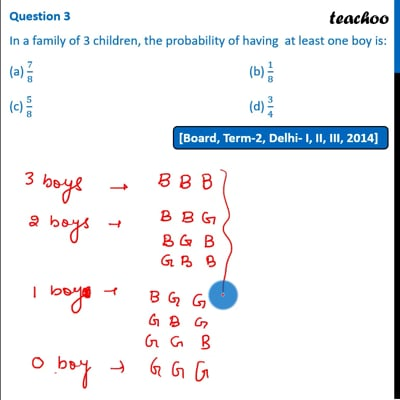Past Year MCQ

Chapter 15 Class 10 Probability
Serial order wise

## (c) 5/8                                                          (d) 3/4This video is only available for Teachoo black users

Maths Crash Course - Live lectures + all videos + Real time Doubt solving!

### Transcript

Question 3 In a family of 3 children, the probability of having at least one boy is: (a) 7/8 (b) 1/8 (c) 5/8 (d) 3/4 In a family of 3 children, the possible outcomes are BBB BBG BGB GBB BGG GBG GGB GGG Thus, Total number of outcomes = 8 Number of outcomes with atleast one boy = 7 Now, P(atleast one boy) = (𝑛(𝐴))/(𝑛(𝑆)) = 7/8 So, the correct answer is (a) Thus, Total number of outcomes = 8 Number of outcomes with atleast one boy = 7 Now, P(atleast one boy) = (𝑁𝑢𝑚𝑏𝑒𝑟 𝑜𝑓 𝑜𝑢𝑡𝑐𝑜𝑚𝑒𝑠 𝑤𝑖𝑡ℎ 𝑎𝑡𝑙𝑒𝑎𝑠𝑡 1 𝑏𝑜𝑦)/(𝑇𝑜𝑡𝑎𝑙 𝑛𝑢𝑚𝑏𝑒𝑟 𝑜𝑓 𝑜𝑢𝑡𝑐𝑜𝑚𝑒𝑠) = 𝟕/𝟖 So, the correct answer is (a)